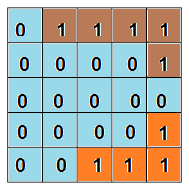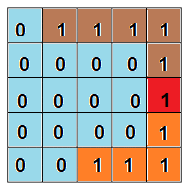Problem title
Difficulty
Avg time to solve

Sort Big List Dates
Moderate
20 mins
Find All Subsets
Easy
15 mins
Easy
--
Valid Pallindrome ll
Easy
--
Attend Maximum Parties
Moderate
--
Duplicate Characters
Easy
25 mins
Make It Palindrome
Easy
25 mins
Second Most Repeated Word
Easy
25 mins
Sentence Sort
Easy
--
Postorder Traversal
Easy
--3

# Shortest Bridge

Difficulty: MEDIUM
Avg. time to solve
35 min
Success Rate
65%

Problem Statement

#### For example#### For example#### Tony is busy assembling all the avengers, so he called you to solve this problem.

##### Input Format
``````The first line of input contains an integer 'T' representing the number of test cases. Then the 'T' test cases are as follows.

The first line of each test case contains two single-spaced integers ‘N’ and ‘M’, representing the number of rows and columns of the 2-D array, respectively.

For the next 'N' lines, each line contains 'M' space-separated integers (0 or 1), where 0 denotes the water and 1 denotes the land.
``````
##### Output Format:
``````For each test case, print the length of the shortest bridge which connects the two islands.

The output for each test case is printed in a separate line.
``````
##### Note:
``````You do not need to print anything, it has already been taken care of. Just implement the given function.
``````
##### Constraints:
``````1 <= T <= 5
1 <= N, M <= 100
0 <= ARR[i][j] <= 1

Where ‘ARR[i][j]’ is the value of the elements of the 2-D array.

Time Limit: 1 sec.
``````
##### Sample Input 1:
``````2
4 4
0 1 0 0
0 0 0 0
0 1 0 0
1 1 1 1
3 3
1 1 0
1 0 0
0 0 1
``````
##### Sample Output 1:
``````1
2
``````
##### Explanation For Sample Input 1:
``````Test Case 1: Cells of the bridge are marked using B.
0 1 0 0
0 B 0 0
0 1 0 0
1 1 1 1

Here the minimum size of the bridge is 1. So, the answer is 1.

Note: Below shown bridge is also a valid bridge but its size is 3 which is not minimum.
0 1 B 0
0 0 B 0
0 1 B 0
1 1 1 1

Test Case 2: Cells of the bridge are marked using B.
1 1 0
1 0 0
B B 1

Here the minimum size of the bridge is 2. So, the answer is 2.
``````
##### Sample Input 2:
``````1
5 5
0 1 1 1 1
0 0 0 0 0
0 0 0 0 0
1 1 1 1 1
1 1 1 1 1
``````
##### Sample Output 2:
``````2
``````
##### Explanation For Sample Input 2:
``````Test Case 1:
Cells of the bridge are marked using B.

0 1 1 1 1
0 0 B 0 0
0 0 B 0 0
1 1 1 1 1
1 1 1 1 1

Here the minimum size of the bridge is 2. So, the answer is 2.
``````Console